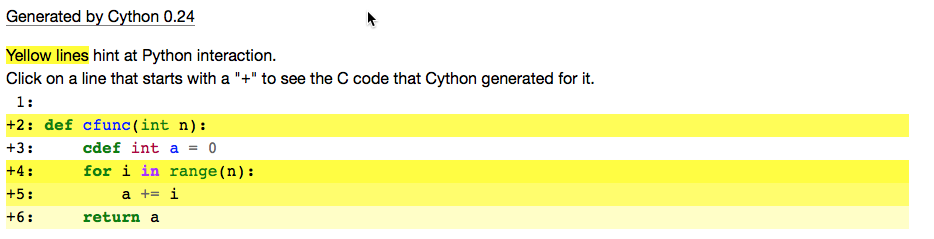# Executing Cython Code

## Overview

Teaching: 20 min
Exercises: 0 min
Questions
• How do I run my Cython program?

• What are the different options for running Cython programs?

Objectives
• Learn the different ways of running Cython programs.

### Manual Compiliation

Cython code is normally saved in files ending with `.pyx` (the x indicates it is different from standard Python code.) A Cython file is be translated to C using the command:

``````cython my_module.pyx
``````

This will create a file called `my_module.c` which is the C source for a Python extension module. A useful additional switch is `-a` which will generate an HTML document (`my_module.html`) that shows which Cython code translates to which C code line by line.

Once the C file has been generated, it must be compiled into a shared library. This may vary according to the operating system, but for Linux it would be something like:

``````gcc -shared -pthread -fPIC -fwrapv -O2 -Wall -fno-strict-aliasing -I/usr/include/python2.7 \
-o my_module.so my_module.c
``````

This command will create a library called `my_module.so`. This library can be treated just like any Python module and imported using the normal import statement:

``````import my_module
``````

### A Simpler Way

Cython can be used conveniently and interactively from a web browser through the IPython notebook.

To enable support for Cython compilation, install Cython and load the Cython extension from within IPython:

``````%load_ext Cython
``````

Cython code can now be compiled using the `%%cython` cell magic command:

``````%%cython
​
def cfunc(int n):
cdef int a = 0
for i in range(n):
a += i
return a

print cfunc(10)
``````

It is also possible to see Cython’s code analysis using the `--annotate` option.

``````%%cython --annotate
​
def cfunc(int n):
cdef int a = 0
for i in range(n):
a += i
return a
``````

This generates an annotated list of the code:### A Simple Example

The following pure Python example generates a list of kmax prime numbers.

``````def primes(kmax):
p = [None] * 1000 # Initialize the list to the max number of elements
if kmax > 1000:
kmax = 1000
result = []
k = 0
n = 2
while k < kmax:
i = 0
while i < k and n % p[i] != 0:
i = i + 1
if i == k:
p[k] = n
k = k + 1
result.append(n)
n = n + 1
return result

%timeit primes(1000)
``````

Timing this generates the following result:

``````10 loops, best of 3: 83.7 ms per loop
``````

The same code can be run without any changes in Cython. The simplest way to do this is by using the `%%cython` cell magic:

``````%%cython
def primes(kmax):
p = [None] * 1000 # Initialize the list to the max number of elements
if kmax > 1000:
kmax = 1000
result = []
k = 0
n = 2
while k < kmax:
i = 0
while i < k and n % p[i] != 0:
i = i + 1
if i == k:
p[k] = n
k = k + 1
result.append(n)
n = n + 1
return result

%timeit primes(1000)
``````

Here’s the result:

``````10 loops, best of 3: 54.3 ms per loop
``````

As you can see, this improved the performance of the pure Python implementation. But can we do better? Let’s try adding some types. Uncomment the lines below to see how this affects the execution.

``````%%cython
def primes(int kmax):
cdef int i, k, n
cdef int p
if kmax > 1000:
kmax = 1000
result = []
k = 0
n = 2
while k < kmax:
i = 0
while i < k and n % p[i] != 0:
i = i + 1
if i == k:
p[k] = n
k = k + 1
result.append(n)
n = n + 1
return result

%timeit primes(1000)
``````

Now we see the following times:

``````1000 loops, best of 3: 1.88 ms per loop
``````

Wow, that made a big difference!

### Using `pyximport`

Cython also provides a means of importing Cython programs directly using the `pyximport` module. To use `pyximport`, you must first import and initialize the module, then your programs will be able to use the `import` statement as you would normaly do for a Python module. The `pyxmodule` is included with Cython.

The `pyximport` module is loadeed and initialiazes as follows:

``````import pyximport
pyximport.install()
``````

Your program can the load a Cython module (ending in `.pyx`) using the normal import mechanism:

``````import my_cython_module as mcm
``````

## Key Points

• Cython programs can be compiled manually.

• Cython provides support for IPython to compile and run Cython programs.

• The pyximport module allows importing Cython programs directly.